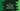# C program to print the current time, day, month and year### C program to print the current time, day, month, and year :

In this C programming tutorial, we will learn how to print the current time, day, month, and year. This program will use time.h header file to print these values. The logic required to print these values is already implemented. You need to import time.h header file to use these features. We will use the following values in this example :

1.tm_mday : It indicates the day of the month. Its range is from 1 to 31. 2.tm_mon : It indicates the month ranging from 0 to 11. 3.tm_year : It indicates the number of the current year starting from 1900. So, to print the actual value of the current year, you will have to add 1900 to its value. 4.tm_wday : Day of the week. The range is from 0 to 6. 5.tm_yday : the day in a year and the range is from 0 to 365.

We will use the above variables in our example program below :

### C program :

``````#include <stdio.h>
#include <time.h>

int main()
{
time_t s, val = 1;
struct tm* current_time;

s = time(NULL);

current_time = localtime(&s);

printf("Day of the month = %d\n",current_time->tm_mday);
printf("Day in this year = %d\n",current_time->tm_yday);
printf("Day in this week = %d\n",current_time->tm_wday);
printf("Month of this year = %d\n",(current_time->tm_mon + 1));
printf("Current year = %d\n",(current_time->tm_year + 1900));
printf("hour:min:sec = %02d:%02d:%02d\n",
current_time->tm_hour,
current_time->tm_min,
current_time->tm_sec);

return 0;
}
``````

### Output :

If you run this program, it will print the following output :

``````Day of the month = 22
Day in this year = 80
Day in this week = 4
Month of this year = 3
Current year = 2018
hour:min:sec = 20:26:18
``````

One thing you can see here that we are adding 900 to the value of the current year because this value gives us the number of years starting from 900. Similarly, to print the exact value of the current month, e.g. current month at this time of writing this blog is March and so we are printing 3, we are adding 1 to the result value. Because its range starts from 0.